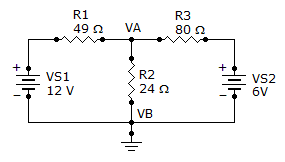# Electrical Engineering - Branch, Loop and Node Analyses - Discussion

Discussion Forum : Branch, Loop and Node Analyses - General Questions (Q.No. 11)
11.
What is the current through R2?177 mA
250 mA
500 mA
125 mA
Explanation:
No answer description is available. Let's discuss.
Discussion:
9 comments Page 1 of 1.

Karthik Netha said:   2 years ago
Nice explanation, thanks @Antony.

P.k.r said:   4 years ago
But, the current through R2 is 0.040 @Antony.

Maraia said:   6 years ago
Good explanation @Antony.

Antony said:   6 years ago
Even using the super-position theorem, the result remains the same. But we need to apply it with care.
Lets, take the first source, 12V alone and put a short across the 6V supply.
Now, calculate the effective resistance of the circuit.
Eff Res. = 49 + (80*24)/(80+24)
= 67.17 ohm.
Now total current supplied by the source 12V = 12/67.17
= 0.178A.

The current through the 24-ohm resistor (using current divider rule) = main current * resistance of other branch / total resistance.

ie. = 0.178 * 80/(80+24).
= 0.137A ----------------- current through R2 by source 12V.

Now take the same using 2nd source, 6V.

Eff. res. = 49*24/(49+24) + 80.
= 96.109 ohm.

Total current supplied by the 6V source = 6/96.109.
= 0.06 A.

current through R2 resistor = 0.06*49/(49+24).
= 0.040A ---- current through R2 by the source 6 V.

Now add the individual source currents to obtain the resultant current through the R2 resistor = 0.137 + 0.40.
= 0.177A.

@Nawal Kumar.

Antony said:   6 years ago
Hi all. The sign convention is somewhat a big deal in deciding the answer.
First of all, define the nodes with one as the reference node. Then try to apply KCL at the node where the unknown parameter exists.
* As per conventional rules, the current incoming is considered negative and outgoing is positive to the node.
Now assume the unknown node voltage as the biggest one, so the assumed current would flow away from the node. And here we begin to write the equation.
At the node under consideration,

(VA-12) VA (VA-6)
-------- +----- + ------ = 0
49 24 80

Same as the equation @Mallesh.

After solving the equation you will get the Va value as 4.29V and the current value as 178mA.

@All.
You might have a doubt about the (Va-12)Volts or the (Va-6)Volts, we just have assumed that voltage at Va is the biggest, so the current would flow away from the node. And the current leaving from a node is considered to be positive.

Ekanath said:   8 years ago
Please can anyone solve complete equation.
(1)

Nawal kumar ray said:   8 years ago
By applying superposition theorem.

First 12 volt active and 6 volt replaced by short circuit.

(24*80)/(24+80) = 18.47 ohm.

Current in equation 1.

I1 = 12/(18.47+49) = 0.25 Amp.

Take 6 volt with 12 volt short circuit.

Required v = 49/(49+24)+80 = 80.7 ohm.

I2 = 6/80.7 = 0.074 Amp.

Total current across R2 = 0.25+0.074 = 0.324 Amp.

By applying node analysis

`(VA-12)    VA     (VA-6)-------- +----- + ------  = 0     49        24      80`

By solving Va = 4.289 v

I thr r2 = 4.289/24 = 178 mA